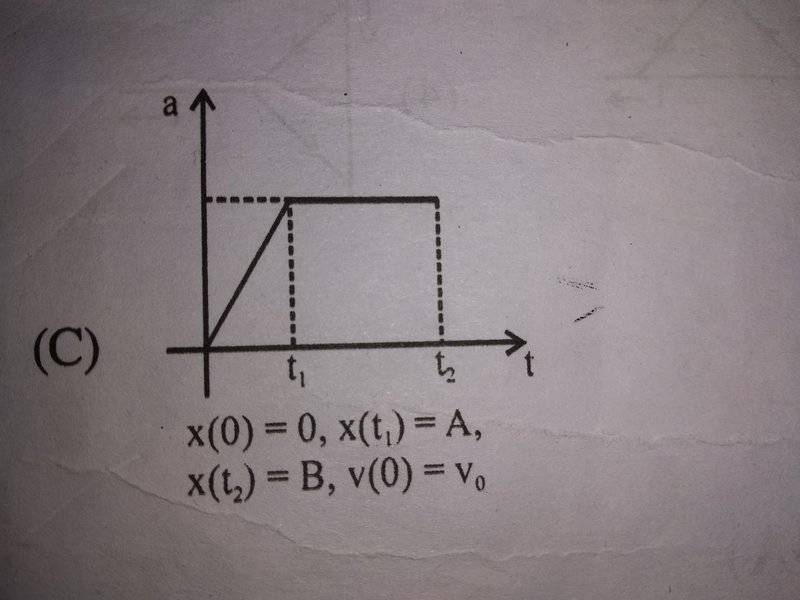# Condition for Instantaneous Acceleration = Average Acceleration

Gold Member

## Homework Statement[/B]
Look at this graph.Is Instantaneous Acceleration=Average Acceleration here?
What should be done to check whether Average Acceleration=Instantaneous Acceleration?

## Homework Equations

Average Acceleration motion equations

## The Attempt at a Solution

I know that Average Acceleration = (Final Velocity-Initial Velocity)/Time Interval
I can't proceed further.
I will be thankful for any help!

#### Attachments

Homework Helper
Gold Member
2021 Award
When a quantity is changing continuously within a time interval its average value is equal to its instantaneous value only once. When a quantity is constant over a time interval, its average value is always equal to its instantaneous value. Does that help? Read the graph.

On edit: Also see correction below.

Last edited: